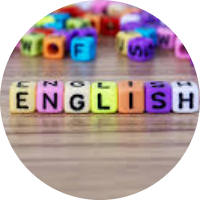# Expressions, Equations and Functions

This set of MCQs helps you brush up on important math topics and prepare you to dive into skill practice.

Start Quiz

Evaluate 8a - b, if a = 10   and   b = 6.

74

1000

100

84

7+{2(6-4)}  Evaluate the expression.

-11

15

11

-15

Translate this phrase into an algebraic expression.
8 less than the product of 4 and a number
Use the variable m to represent the unknown number.

4m + 8

8m -4

4m - 8

8m +4

Determine whether the given value of the variable is a solution.
x + 6 = 14, when x = 8.

Yes

No

Translate the verbal phrase into an expression. "3 less than the square of a number p".

-p^2/3

p^2−3

p^2+3

p^2/3

Is –462 ÷ –11 positive or negative?

Yes

No

Subtract : (3y^2+4y+4)–(4y+3)

3y^2+9

-3y^2+1

3y^2-1

3y^2+1

Factor x^4+12x^2+36 completely.

(x^2-6)

(x^2+6)^2

(x^2-6)^2

(x^2+6)

Quiz/Test Summary
Title: Expressions, Equations and Functions
Questions: 8
Contributed by: